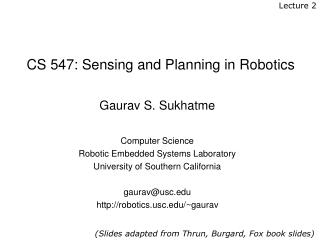DownloadDownload PresentationCS 547: Sensing and Planning in Robotics

# CS 547: Sensing and Planning in Robotics

Download Presentation## CS 547: Sensing and Planning in Robotics

- - - - - - - - - - - - - - - - - - - - - - - - - - - E N D - - - - - - - - - - - - - - - - - - - - - - - - - - -
##### Presentation Transcript

1. Lecture 2 CS 547: Sensing and Planning in Robotics Gaurav S. Sukhatme Computer Science Robotic Embedded Systems Laboratory University of Southern California gaurav@usc.edu http://robotics.usc.edu/~gaurav (Slides adapted from Thrun, Burgard, Fox book slides)

2. Bayes Filters: Framework • Given: • Stream of observations z and action data u: • Sensor modelP(z|x). • Action modelP(x|u,x’). • Prior probability of the system state P(x). • Wanted: • Estimate of the state X of a dynamical system. • The posterior of the state is also called Belief:

3. Recursive Bayesian Updating Markov assumption: znis independent of d1,...,dn-1if we know x.

4. Markov Assumption Underlying Assumptions • Static world • Independent noise • Perfect model, no approximation errors

5. Recursive Bayesian Updating • Action Update

6. Bayes Filter Algorithm • Algorithm Bayes_filter( Bel(x),d ): • h=0 • Ifd is a perceptual data item z then • For all x do • For all x do • Else ifd is an action data item uthen • For all x do • ReturnBel’(x)

7. Discrete Bayes Filter Algorithm • Algorithm Discrete_Bayes_filter( Bel(x),d ): • h=0 • Ifd is a perceptual data item z then • For all x do • For all x do • Else ifd is an action data item uthen • For all x do • ReturnBel’(x)

8. Bayes Markov Total prob. Markov Markov z = observation u = action x = state Bayes Filters

9. Bayes Filters are Familiar! • Kalman filters • Particle filters • Hidden Markov models • Dynamic Bayesian networks • Partially Observable Markov Decision Processes (POMDPs)

10. Summary • Bayes rule allows us to compute probabilities that are hard to assess otherwise. • Under the Markov assumption, recursive Bayesian updating can be used to efficiently combine evidence. • Bayes filters are a probabilistic tool for estimating the state of dynamic systems.